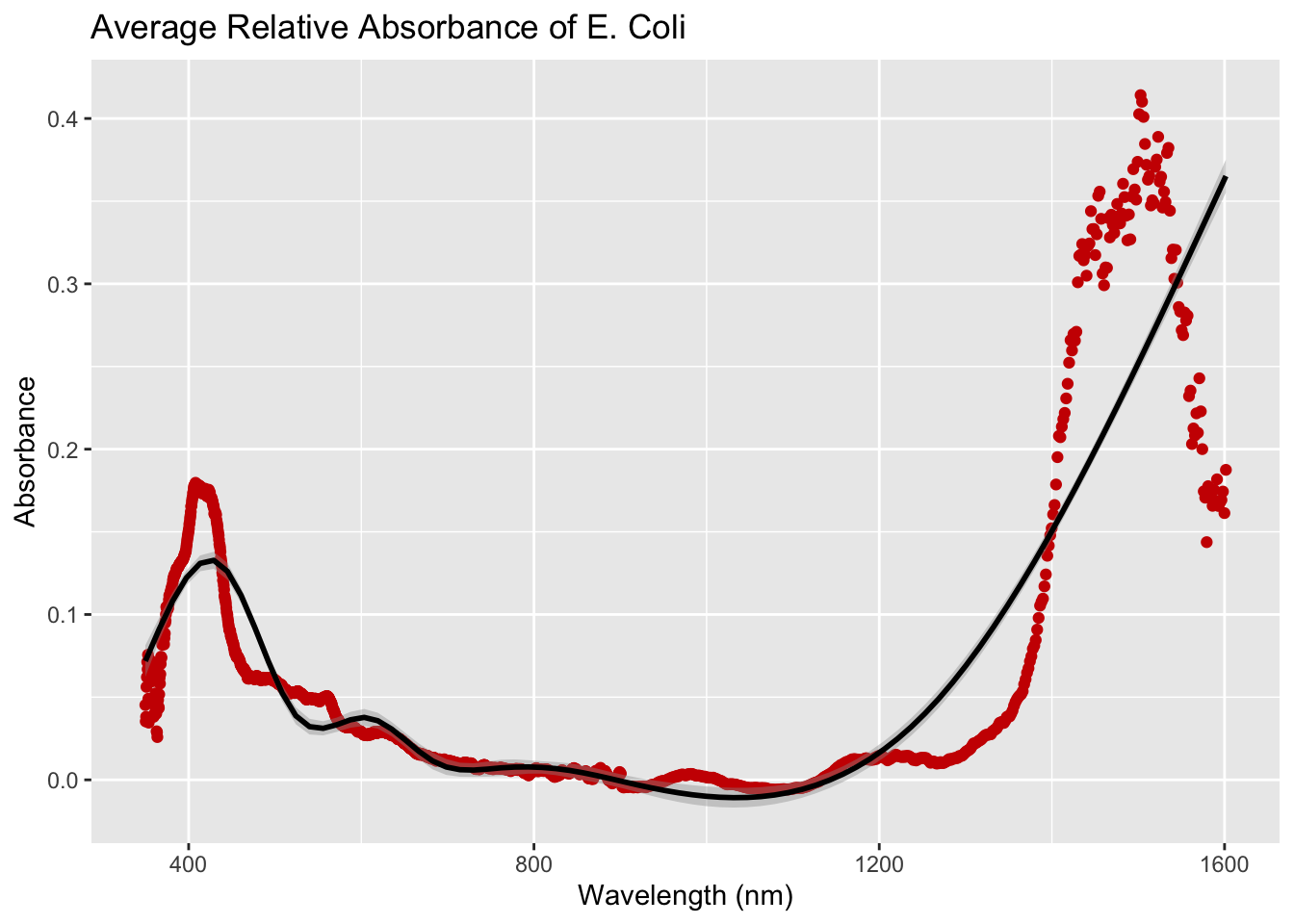# Using dplyr and foreach to read in multiple data sets from disk

I had a student (Jack Fogliasso) bring me a problem from a Microbiology lab where they are trying to identify bacteria using lasers. One of the items they wanted to understand was the absorption rates of different wavelengths. So they have a machine to do the lasering and it reads out a text file that of course has a ton of meta data in it, is semi-colon delimited, and there’s one file per trial. So not the standard situation that one might encounter in a basic applied statistics class.

Jack and I talked about automation and reproducibility and how R is great for tasks like this, and he got to work. What is shown below is an effective use of `dplyr` and to read in a data set from disk, do some processing, and create a visualization. This process can be expanded to any number of files thanks to the efficient looping of `foreach`, and the combination of `dplyr` and `ggplot2` can accommodate different file names, such as for different new bacteria.

# System setup

This example uses the `ggplot2`, `dplyr` and `foreach` packages.

``````knitr::opts_chunk\$set(echo = TRUE, warning=FALSE, message=FALSE)
library(ggplot2)
library(dplyr)
library(foreach)``````

# Data import

Read in the data for the three experimental trials.

1. get all .txt files in the working directory and put into a list.
``````list_files <- list.files(pattern = ".txt", full.names = TRUE)
list_files``````
``##  "./Ec1.txt" "./Ec2.txt" "./Ec3.txt"``
1. Use the `%do%` function in the `foreach` package to efficiently loop over all items in the list. This read in the data using `read.delim`, turns it into a data frame, so that we can add the file name to the data set before combining each data set using `rbind` (stacking or concatonating).
``````eColi <- foreach(i=1:length(list_files), .combine=rbind) %do% {
data.frame() %>%
mutate(file=gsub(".txt", "", list_files[[i]]))# add an identifier to index each trial
}
``````##   Pixel Wavelength    Dark Reference Raw.data..1 Dark.Subtracted..1 X.TR..1
## 1     0     349.84 1161.60   1266.60     1257.96              96.36 91.7714
## 2     1     350.27 1160.72   1263.56     1258.04              97.32 94.6324
## 3     2     350.71 1161.20   1262.84     1259.56              98.36 96.7729
## 4     3     351.14 1158.96   1264.04     1256.76              97.80 93.0719
## 5     4     351.58 1150.24   1259.64     1252.12             101.88 93.1261
## 6     5     352.01 1144.08   1256.92     1243.72              99.64 88.3020
##   Absorbance..1 Raw.data..2 Dark.Subtracted..2 X.TR..2 Absorbance..2
## 1        0.0373           0                  0       0             0
## 2        0.0240           0                  0       0             0
## 3        0.0142           0                  0       0             0
## 4        0.0312           0                  0       0             0
## 5        0.0309           0                  0       0             0
## 6        0.0540           0                  0       0             0
##   Raw.data..3 Dark.Subtracted..3 X.TR..3 Absorbance..3 Lamp.FitCurve
## 1           0                  0       0             0             0
## 2           0                  0       0             0             0
## 3           0                  0       0             0             0
## 4           0                  0       0             0             0
## 5           0                  0       0             0             0
## 6           0                  0       0             0             0
## 1                0                      NA                       0
## 2                0                      NA                       0
## 3                0                      NA                       0
## 4                0                      NA                       0
## 5                0                      NA                       0
## 6                0                      NA                       0
## 1                       0 ./Ec1
## 2                       0 ./Ec1
## 3                       0 ./Ec1
## 4                       0 ./Ec1
## 5                       0 ./Ec1
## 6                       0 ./Ec1``````
1. Shorten the names to something reasonable.
``````names(eColi)[c(5:16,19:21)] <- paste0(rep(c("raw", "dark", "X", "abs", "irr"),3), 1:3)
names(eColi)``````
``````##   "Pixel"            "Wavelength"       "Dark"             "Reference"
##   "raw1"             "dark2"            "X3"               "abs1"
##   "irr2"             "raw3"             "dark1"            "X2"
##  "abs3"             "irr1"             "raw2"             "dark3"
##  "Lamp.FitCurve"    "Irradiance.Ratio" "X1"               "abs2"
##  "irr3"             "file"``````

# Data Processing

Create the average absorbance of the 3 trials per wave length.

``````eColi_abs <- eColi %>% group_by(Wavelength) %>% summarise(avgRelAbs = mean(abs1))
``````## # A tibble: 6 x 2
##   Wavelength avgRelAbs
##        <dbl>     <dbl>
## 1       350.    0.0453
## 2       350.    0.0353
## 3       351.    0.0382
## 4       351.    0.0562
## 5       352.    0.0621
## 6       352.    0.0713``````

# Analyzing

Plot the average relative absorbance for the E. coli.

``````ggplot(eColi_abs, aes(x=Wavelength, y=avgRelAbs)) +
geom_point(color="#CC0000") + geom_smooth(color="black") +
labs(title="Average Relative Absorbance of E. Coli",
x="Wavelength (nm)", y="Absorbance")``````